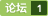### matlab的多目标遗传算法 [问题点数：20分]Matlab多目标遗传算法 （附带程序）

Matlab 多目标优化 遗传算法
Matlab <em>多目标</em>优化 <em>遗传算法</em> 源程序 很好的应用案例 基于MATLAB

【 MATLAB 】遗传算法程序

Matlab多目标遗传算法 （附带程序）下载

【搞搞算法】Matlab遗传算法工具箱gatbx小试

matlab遗传算法工具箱的使用

<em>多目标</em><em>遗传算法</em>应用案例，简单易懂易上手。其中还有清晰的案例。
C++实现多目标遗传算法（0/1背包问题）
（背包问题）：背包只能容得下一定重量b的物品，物品有m种，每种物品有自己的重量w(i)和价值v(i)，从这些物品中选择装入背包，是背包不超过重量b，但价值又要最大。 上面为单目标的0/1规划问题，也就是说只考虑物体的重量不考虑物体的体积，形状等问题，一般而言，利用动态规划可以很好地解决背包问题，但是如果物体过多，使用动态规划将浪费很大的资源． <em>遗传算法</em>作经典的人工智能算法，可以很

function MultiGA() %% <em>遗传算法</em>求解<em>多目标</em>优化案例 %% 将原<em>多目标</em>函数改写为f1=(x^2+y^2)/4;f2=x(1-y)+10; % 运用线性叠加法，F=a*f1(x)+b*f2(x)  ,a+b=1 % 总目标函数改写为  f=0.6*(x^2+y^2)/4+0.4*(x*(1-y)+10);   popse=100;  % 种群数目 maxgen=50;

MATLAB多目标遗传算法
NSGA非支配排序<em>遗传算法</em>就是一种以基本<em>遗传算法</em>为基础的<em>多目标</em>寻优策略，因为其在<em>多目标</em>寻优领域的优势，成为人们的研究热点。

<em>遗传算法</em>简介<em>遗传算法</em>（Genetic Algorithms）是目前适用比较广泛的随机搜索和优化算法，也是众所周知的进化计算方法。在本文中，我们就将利用GA求解一个简单的无约束线性规划问题来理解<em>遗传算法</em>到底是如何工作的。
Matlab遗传算法优化问题求解的示例代码
Matlab的<em>遗传算法</em>实现，可作为进一步改进的框架

<em>遗传算法</em>实现<em>多目标</em>优化，有约束条件的！用C语言实现的@
matlab求解多目标遗传算法代码

<em>多目标</em><em>遗传算法</em>工具箱，里面有相关的代码，可用于优化代理模型寻优，分享出来

这一部分主要讲解如何写自己的目标函数。什么事目标函数呢？你使用<em>遗传算法</em>工具箱主要是想找到某一个函数的最优解吧，那么这个函数就是目标函数。这个函数你必须写成一个M文件的形式。这样才能符合<em>遗传算法</em>工具箱的要求，当然要求不仅仅这些，还有下面的一些要求：     该函数应该接受一个矢量作为输入参数，该矢量的长度应该等于目标函数中独立变量的个数，该函数应该返回一个标量，这个标量应该是这个输入参数相对应
【搞搞算法】用遗传算法解决多目标规划问题_论文阅读笔记

<em>多目标</em><em>遗传算法</em>NSGA因所读的一篇论文中，为了解决<em>多目标</em>的最优解问题，作者使用了一种称为NSGA-II(Improved Non-dominated Sorting Genetic Algorithm)的<em>遗传算法</em>，花了两天时间了解下，此为何物。其中NSGA以及NSGA-II的原理说明内容大部分取自2008年李莉的硕士论文《基于<em>遗传算法</em>的<em>多目标</em>寻优策略的应用研究》，故将此文定为转载。首先需要了解一种称
Matlab多目标遗传算法工具箱

<em>遗传算法</em>求解<em>多目标</em>问题，其中将<em>多目标</em>问题转化为加权的单目标问题
Python遗传算法框架使用实例（二）多目标优化问题Geatpy for Python与Matlab的对比学习

<em>遗传算法</em> 建立GeneticAlgorithm.py import numpy as np from GAIndividual import GAIndividual import random import copy import matplotlib.pyplot as plt class GeneticAlgorithm: ''' The class for...

NSGA-2 多目标优化 遗传算法 MATLAB

MultiObjGA Code(多目标数值优化遗传算法源代码)

MATLAB（1）基于遗传算法解决最优化问题及相应的MATLAB遗传工具箱使用
MATLAB（1）基于<em>遗传算法</em>解决最优化问题及相应的MATLAB遗传工具箱使用      摘要：本文将简明扼要的介绍一下<em>遗传算法</em>，并以一个简单的二元一次方程组求解为例，演示用MATLAB工具箱快捷地实现<em>遗传算法</em>。      对于取最小值的最优化问题，<em>遗传算法</em>借鉴生物遗传现象使具有一定数量的候选解的种群向更好的解进化，该方法是通过种群进化，使得适应度函数代入估计参数后达到最值

<em>多目标</em><em>遗传算法</em>研究

NSGA-II 多目标遗传算法
NSGA-II <em>多目标</em><em>遗传算法</em>MATLAB实现，实测可以运行，可以参考

<em>多目标</em><em>遗传算法</em>的几个程序，希望对大家有用哦
Ansys遗传算法优化实例

NSGA-II matlab 遗传算法源码
NSGA-II <em>matlab</em> <em>遗传算法</em>源码 不错

（1）初识<em>遗传算法</em>      <em>遗传算法</em>，模拟达尔文生物进化论的自然选择和遗传学机理的生物进化过程的计算模型，一种选择不断选择优良个体的算法。谈到遗传，想想自然界动物遗传是怎么来的，自然主要过程包括染色体的选择，交叉，变异（不明白这个的可以去看看生物学），这些操作后，保证了以后的个体基本上是最优的，那么以后再继续这样下去就可以一直最优了。 （2）解决的问题 先说说自己要解决的问题吧。<em>遗传算法</em>很

NSGA2优化算法Matlab求解多目标优化问题
NSGA2优化算法Matlab求解<em>多目标</em>优化问题，<em>遗传算法</em>优化+帕累托排序，有效地解决了<em>多目标</em>优化问题，算例可行有效。

<em>遗传算法</em>求<em>多目标</em>函数最优解得两种方法理想点法和线性加权法

A structure MATLAB implementation of MOPSO for Evolutionary Multi-Objective Optimization A structured MATLAB implementation of MOEA/D for Evolutionary Multi-Objective Optimization

MATLAB遗传算法VRP
MATLAB<em>遗传算法</em>单车场单车型有容量约束的多车VRP问题，解压后直接运行

<em>遗传算法</em>的概念最早是由Bagley J.D 于1967年提出的。后来Michigan大学的J.H.Holland教授于1975年开始对<em>遗传算法</em>（Genetic Algorithm, GA）的机理进行系
matlab遗传算法求最优解

<em>遗传算法</em>就是在一定的自变量有限的取值范围内，随机取若干个个体，每个个体相当于自变量范围内的一个取值，若干个个体共同组成一个种群，个体对于环境的适应能力体现为该个体对应的因变量，不同的个体得到的结果不同，对于结果较好的个体，其下一代在种群中的占比更高，对于结果不好的个体，其下一代在种群中的占比会更少，简单来说，就是好的个体被保留，坏的个体被淘汰。经过不断的更新换代，最后结果会不断逼近最优的结果。 ...

<em>多目标</em>perota优化MATLAB算法，运用算法，通过MATLAB进行自动优化
MATLAB求多目标优化问题——fminimax
MATLAB求<em>多目标</em>优化问题使用fminimax求解，包括模型、fminimax函数说明以及实例。

<em>遗传算法</em>简单介绍与MATLAB实现（二）上一篇文章中我们简单的介绍了一了一下<em>遗传算法</em>，其中提到了多元函数f=f(x,y)f=f(x, y).所以在这里我们就定义一个二元函数，作为第一个练手的程序。并以此作为示例进行编程示范。

1.简单介绍 略过<em>遗传算法</em>的发展史，本文直接讲解<em>遗传算法</em>的基本原理与实现。在生活中很多问题都可以转换为函数优化问题，大部分函数优化问题都可以写成求最大值或者最小值的形式，本文我们就用<em>遗传算法</em>来求一元函数最大值（最小值问题可以转化为最大值）。 <em>遗传算法</em>就是先产生出一定数量的个体，通过繁衍，交叉，变异产生更多的个体，对这些新的个体按一定条件进行筛选，始终留下一定数量的个体，如此反复，便能有最优解出...

<em>遗传算法</em>程序（一）:    说明: fga.m 为<em>遗传算法</em>的主程序; 采用二进制Gray编码,采用基于轮盘赌法的非线性排名选择, 均匀交叉,变异操作,而且还引入了倒位操作! function [BestPop,Trace]=fga(FUN,LB,UB,eranum,popsize,pCross,pMutation,pInversion,options) % [BestPop,Trace]=...
MATLAB中自带遗传算法函数GA的用法
ga 用<em>遗传算法</em>寻找函数的最优解 语法规则 x = ga(fitnessfcn,nvars) x = ga(fitnessfcn,nvars,A,b) x = ga(fitnessfcn,nvars,A,b,Aeq,beq) x = ga(fitnessfcn,nvars,A,b,Aeq,beq,LB,UB)% 其中fitnessfc为函数的句柄或者为匿名函数
【搞搞算法】多目标遗传算法NSGA-II的C语言代码使用手册

MATLAB R2018a 全局优化工具箱学习（一）遗传算法
Global Optimization Toolbox   在MATLAB R2018a 中发现了全局优化的工具箱以及增加的数据和机器学习工具箱，对于求解全局最优值很是方便。 MATLAB Global Optimization Toolbox 学习： 全局优化工具箱提供了一些函数, 用于寻找包含多个极大值或极小值的问题的全局解。工具箱包括全局搜索、多初始点、模式搜索、<em>遗传算法</em>、<em>多目标</em>...

matlab遗传算法gatbx工具箱介绍及安装说明

-

<em>遗传算法</em>解决全局优化（即为最值点如图中C，D），而局部最优解决的是极值点问题（如图中A，B） 1.       <em>遗传算法</em>流程；   [plain] view plain copy %<em>遗传算法</em>的伪代码描述：   %Procedure GA   %Begin   %       T=0;   %       Initial